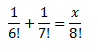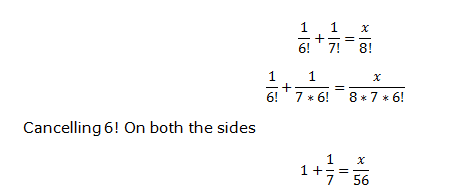# NCERT Solution for Permutation and Combinations of Class 11 Chapter 7 Exercise 7.2

Question 1:
Evaluate
1. 8!
2.  4! – 3!
Question 2:
Is 3! + 4! = 7!?
Question 3:
Compute
8!/(6!X 2!)
Question 4:
Calculate x ifQuestion 5:
Evaluate,
n!/(n-r)!
when
(i) n = 6, r = 2
(ii) n = 9, r = 5

Solution 1
We know that
n!=n(n-1)(n-2)……3*2*1
So solving the below questions based on that
1. 8! = 1 × 2 × 3 × 4 × 5 × 6 × 7 × 8 = 40320
2. First calculate  the factorials 4! = 1 × 2 × 3 × 4 = 24
3! = 1 × 2 × 3 = 6
Then 4! – 3! = 24 – 6 = 18
Solution 2
We know that factorials is given by
n!=n(n-1)(n-2)……3*2*1
So solving the below questions based on that
3! = 1 × 2 × 3 = 6
4! = 1 × 2 × 3 × 4 = 24
3! + 4! = 6 + 24 = 30
7! = 1 × 2 × 3 × 4 × 5 × 6 × 7 = 5040
So it is clear that
3! + 4! ≠ 7!
Solution 3
8!/(6!X 2!)
= 8*7 *6!/6! * 2
=8*7/2=28
Solution 4:Solving this
x=64
Solution  5:
n!/(n-r)!
when
(i) n = 6, r = 2
(ii) n = 9, r = 5
n!/(n-r)!= 6!/4!=30
n!/(n-r)!= 9!/4!=9*8*7*6*5=15120